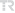## Testing distributional assumption of unit-Lindley regression model

HACETTEPE JOURNAL OF MATHEMATICS AND STATISTICS, vol.51, no.3, pp.882-899, 2022 (SCI-Expanded)• Publication Type: Article / Article
• Volume: 51 Issue: 3
• Publication Date: 2022
• Doi Number: 10.15672/hujms.932811
• Journal Name:
• Journal Indexes: Science Citation Index Expanded (SCI-EXPANDED), Scopus, zbMATH, TR DİZİN (ULAKBİM)
• Page Numbers: pp.882-899
• Keywords: &nbsp, Chi-square test, deviance test, power of test, smooth test, unit-Lindley distribution, parametric bootstrap, BETA REGRESSION, SMOOTH TESTS, NORMALITY
• Gazi University Affiliated: Yes

#### Abstract

This paper proposes smooth goodness of fit test statistic and its components to test the distributional assumption of the unit-Lindley regression model, which is useful for describing data measured between zero and one. Orthonormal polynomials on the unit-Lindley distribution, score functions and Fisher???s information matrix are provided for the smooth test. Deviance and Pearson???s chi-square tests are also adapted to the unit-Lindley regression model. A parametric bootstrap simulation study is conducted to compare type I errors and powers of the tests under different scenarios. Empirical findings demonstrate that the first smooth component, deviance, and chi-square tests have undesirable behavior for the unit-Lindley regression model. A real data set is analyzed by using the developed tests to show the adequacy of the unit-Lindley regression model. Model selection criteria and residual analysis prove that the unit-Lindley regression model provides a better fit than the Beta and simplex regression models for the real data set. Mathematics Subject Classification (2020). 62G10, 62F40